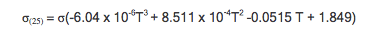# Electrical Conductivity of Soil as a Predictor of Plant Response (Part 2)

Salt in soil comes from the fertilizer we apply but also from irrigation water and dissolving soil minerals.  If more salt is applied in the irrigation water than is leached or taken off in harvested plants, the soil becomes more saline and eventually ceases to support agricultural production (see part 1).  This week, learn an effective way to measure electrical conductivity (EC) in soil.

## How to Measure Electrical Conductivity of the Soil Solution

As mentioned above, the earliest measurements of solution conductivity were made on soil samples, but it was found to be more reliable to extract the soil solution and make the measurements on it. When values for unsaturated soils are needed, those are calculated based on the saturation numbers and conjecture about how the soil dried to its present state. Obviously a direct measurement of the soil solution conductivity would be better if it could be made reliably.

Two approaches have been made to this measurement. The first uses platinum electrodes embedded in ceramic with a bubbling pressure of 15 bars. Over the plant growth range the ceramic remains saturated, even though the soil is not saturated, allowing a measurement of the solution in the ceramic. As long as there is adequate exchange between the ceramic and the soil solution, this measurement will be the EC of the soil solution, pore water EC.

The other method measures the conductivity of the bulk soil and then uses empirical or theoretical equations to determine the pore water EC. The ECH2O 5TE uses the second method. It requires no exchange of salt between soil and sensor and is therefore more likely to indicate the actual solution electrical conductivity. The following analysis shows one of several methods for determining the electrical conductivity of the saturation extract from measurements of the bulk soil electrical conductivity.

Mualem and Friedman (1991) proposed a model based on soil hydraulic properties. It assumes two parallel conduction paths: one along the surface of soil particles and the other through the soil water. The model isEquation 1

Here σb is the bulk conductivity which is measured by the probe, σs is the bulk surface conductivity, σw is the conductivity of the pore water, θ is the volumetric water content, θs is the saturation water content of the soil and n is an empirical parameter with a suggested value around 0.5. If, for the moment, we ignore surface conductivity, and use eq. 1 to compute the electrical conductivity of a saturated paste (assuming n = 0.5 and θs = 0.5) we obtain σb = 0.35σw. Obviously, if no soil were there, the bulk reading would equal the electrical conductivity of the water. But when soil is there, the bulk conductivity is about a third of the solution conductivity. This happens because soil particles take up some of the space, decreasing the cross section for ion flow and increasing the distance ions must travel (around particles) to move from one electrode of the probe to the other. In unsaturated soil these same concepts apply, but here both soil particles and empty pores interfere with ion transport, so the bulk conductivity becomes an even smaller fraction of pore water conductivity.When water evaporates at the soil surface, or from leaves, it is pure, containing no salt, so evapotranspiration concentrates the salts in the soil.

Our interest, of course, is in the pore water conductivity. Inverting eq. 1 we obtainEquation 2

In order to know pore water conductivity from measurements in the soil we must also know the soil water content, the saturation water content, and the surface conductivity. The 5TE measures the water content. The saturation water content can be computed from the bulk density of the soilEquation 3

Where ρb is the soil bulk density and ρs is the density of the solid particles, which in mineral soils is taken to be around 2.65 Mg/m3 . The surface conductivity is assumed to be zero for coarse-textured soil. Therefore, using the 5TE allows us to quantify pore water EC through the use of the above assumptions. This knowledge has the potential to be a very useful tool in fertilizer scheduling.

## Electrical Conductivity is Temperature Dependent

Electrical conductivity of solutions or soils changes by about 2% per Celsius degree. Because of this, measurements must be corrected for temperature in order to be useful. Richards (1954) provides a table for correcting the readings taken at any temperature to readings at 25 °C. The following polynomial summarizes the tablewhere t is the Celsius temperature. This equation is programmed into the 5TE, so temperature corrections are automatic.

## Units of Electrical Conductivity

The SI unit for electrical conductance is the Siemen, so electrical conductivity has units of S/m. Units used in older literature are mho/cm (mho is reciprocal ohm), which have the same value as S/cm. Soil electrical conductivities were typically reported in mmho/cm so 1 mmho/cm equals 1 mS/cm. Since SI discourages the use of submultiples in the denominator, this unit is changed to deciSiemen per meter (dS/m), which is numerically the same as mmho/cm or mS/cm. Occasionally, EC is reported as mS/m or µS/m. 1 dS/m is 100 mS/m or 105 µS/m.

Understanding the difference between electrical conductivity readings in water and in soil can help you make better use of your EC readings. Watch the video to answer questions such as “Why does water that’s 1.9 dS/m not read 1.9 dS/m when it’s in the soil?

### References

Richards, L. A. (Ed.) 1954. Diagnosis and Improvement of Saline and Alkali Soils. USDA Agriculture Handbook 60, Washington D. C.

Rhoades, J. D. and J. Loveday. 1990. Salinity in irrigated agriculture. In Irrigation of Agricultural Crops. Agronomy Monograph 30:1089-1142. Americal Society of Agronomy, Madison, WI.